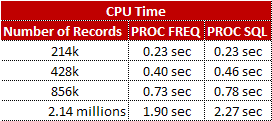# SAS : Count Distinct Values of Variables

This tutorial explains how to count distinct values of variables using PROC SQL and PROC FREQ. We will also check the performance of these two approaches.

PROC SQL : Count Distinct Values
proc sql;
create table t as
select count(distinct make) as n_make, count(distinct type) as n_type
,count(distinct origin) as n_origin
from sashelp.cars;
quit;

PROC FREQ : Count Distinct Values
ods output nlevels = charlevels;
proc freq data=sashelp.cars (keep = make type origin) nlevels;
tables make type origin / nopercent nocol nocum nofreq noprint;
run;
ods output close;

Performance Testing : PROC SQL vs. PROC FREQ

I have stacked raw data set multiple times (500 to 5000 times) to check the performance of these two procedures. In addition, i have added NOPRINT and ODS OUTPUT CLOSE to improve efficiency of the PROC FREQ code.Count Distinct Values of a Variable
%macro stack (inputdata = sashelp.cars, iterations=, out=);

data temp;
length x \$45.;
do i = 1 to &iterations.;
if i then x = "&inputdata.";
output;
end;
drop i;
run;

proc sql noprint;
select x into: n separated by ' '
from temp;
quit;

data &out.;
set &n;
run;

ods output nlevels = charlevels;
proc freq data=outdata (keep = make type origin) nlevels;
tables make type origin / nopercent nocol nocum nofreq noprint;
run;
ods output close;

proc sql;
create table t as
select count(distinct make) as n_make, count(distinct type) as n_type
,count(distinct origin) as n_origin
from outdata;
quit;

%mend;

%stack (inputdata = sashelp.cars, iterations= 500, out= outdata);
%stack (inputdata = sashelp.cars, iterations= 1000, out= outdata);
%stack (inputdata = sashelp.cars, iterations= 2000, out= outdata);
%stack (inputdata = sashelp.cars, iterations= 5000, out= outdata);1.